# Mathematics 311 Solved Assignment 2021-22 | NIOS Senior Secondary Solved Assignment 2021 - 22

## NIOS Senior Secondary Solved Assignment 2021 - 22Mathematics 311 Solved Assignment 2021-22Tutor Marked AssignmentMax Marks: 20

Note:

(i) All questions are compulsory. The marks allotted for each question are beside the questions.

(ii) Write your name, enrollment number, AI name and subject on the top of the first page of the answer sheet.

1. Answer any one of the following questions.       2

(a) In a group of students, 100 students know Hindi, 45 know English and 30 know both. Each of the students knows either Hindi or English. How many students are there in the group? (See Lesson-1)

Ans: Let U be the set of all students in the group.

Let E be the set of all students who know English.

Let H be the set of all students who know Hindi.

HUE=U
Given n (H)=100 and n(E)=45

n(H∩E)=30

Now,

n(U) = n(H)+n(E)−n(H∩E) =100 + 45 – 30

Hence, there are 115 students in the group.

(b) A bag containing 8 red and 5 green balls. Two balls are drawn without replacement (simultaneously) from the bag. Find the probability that both the balls drawn are green. (See Lesson -19)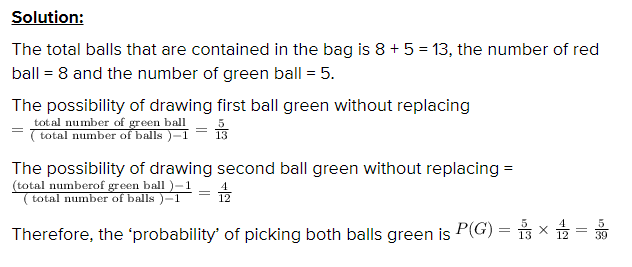2. Answer any one of the following questions.     2

(a) There are 15 boys and 10 girls in your class. A four member committee is to be formed from the students of your class. In how many ways this can be done if the committee consists at least three girls? (See Lesson 6)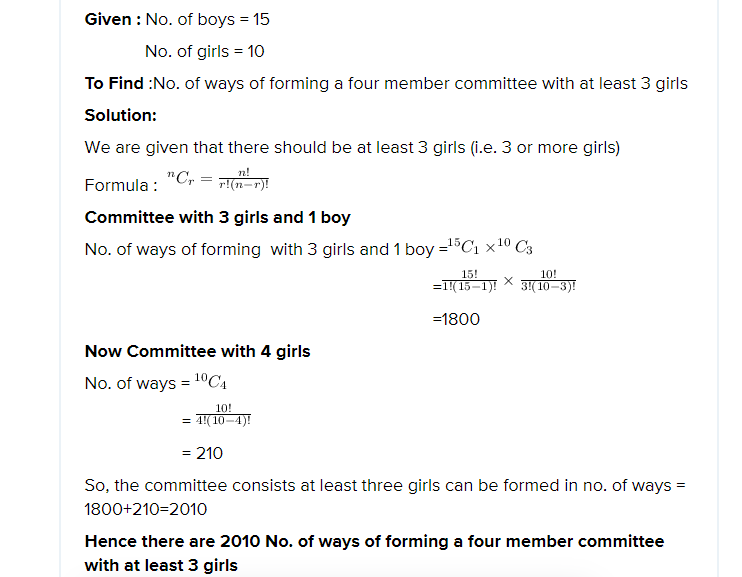(b) If z=1 show that z-1z+1, (z≠-1) is a pure imaginary number. What will you conclude if z = 1? (See Lesson-8)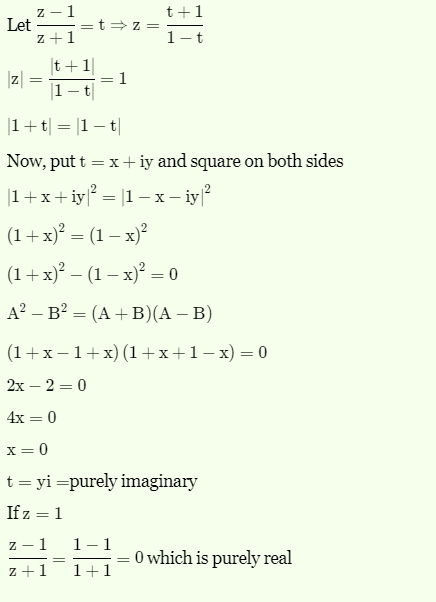3. Answer any one of the following questions.      2

(a) If the ratio of three sides of a triangle is a: b: c = 7:8:9 then show that cos A: cos B: cos C=14: 11: 6. (See Lesson-5)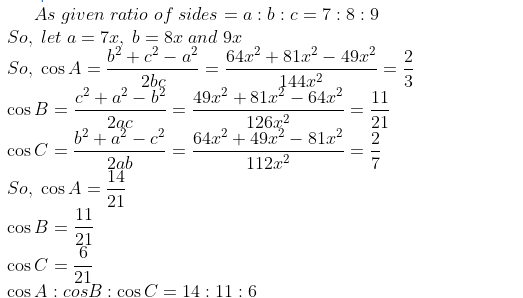(b) Find the radius of the circular section of the sphere x2 + y 2+ z2 = 49 by the plane 2x + 3y – z – 5 14 = 0. (See Lesson-9)

Solution: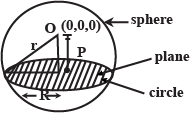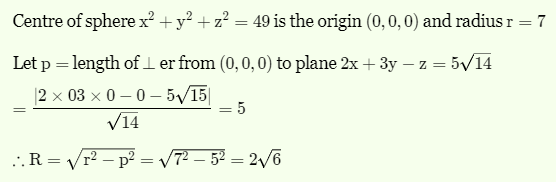4. Answer any one of the following questions.        4

(a) How conic sections are generated. From your surrounding give four examples of each of circle, parabola, ellipse and hyperbola. Prepare a list of their different informations. (See Lesson-12)

Ans: Answer of any one question is necessary

(b) The sum of first three terms of a Geometric Progression is 13/12 and their product is –1. Find Geometric Progression G.P.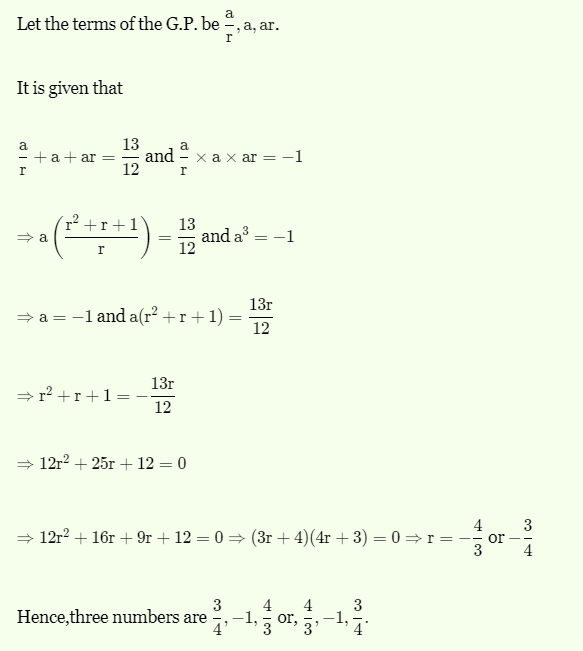5. Answer any one of the following questions.         4

(a)

(i) Give an example of a function whose graph is symmetrical about origin and draw the graph.

(ii) Express 7i 7+ 7i 6+ 3i3 – 2i2 + 1 in the form of a + bi (See Lesson-2 & 8)

Ans: Answer of any one question is necessary

(b) The product of three terms of a GP is 512. If 8 is added to the first term and 6 to the second term, find the new terms form an AP. (See Lesson -6)

Ans: Let a/r, a, ar be the terms in GP.

Then product = (a/r)a(ar) = 512

a3 = 512

So a = 8

(a/r)+8 , a+6, ar are in AP.

8/r + 8, 14, 8r are in AP.

So 28 = 8/r + 8 + 8r

20 = 8/r + 8r

20r = 8+8r2

8r2-20r+8 = 0

2r2-5r+2 = 0

(2r-1)(r-2) = 0

r = ½ or r = 2

When r = ½, the numbers are 16, 8, 4.

When r = 2, the numbers are 4, 8, 16.

6. (a) Prepare any one project of the following projects given below.        6

Observe a one-day cricket match to be held in the year 2016. Prepare a project report indicating the performance and comparison with respect to the following points:

(i) Range of the individual scores of the players.

(ii) Compute team wise mean deviation score.

(iii) Prepare team wise grouped frequency distribution tables, showing the number of over as class intervals and corresponding scores as frequencies.

(iv) Represent the above frequency distribution table (Part iii) with the help of histogram.

(b) Conduct a survey at population in your locality taking a sample of at least 50 families. Prepare a project report with respect to the following points:

(i) Represent the data showing age using class intervals.

(ii) Compute the average income of the families.

(iii) Literacy level of the population in terms of Elementary, Secondary, Senior Secondary, Graduation and above.

(iv) Find the variance of the income of the families.

(v) Represent the data in part (iii) with the help of bar graph.

***